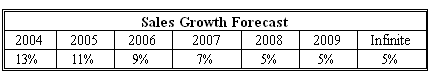### CFA Practice Question

There are 201 practice questions for this study session.

### CFA Practice Question

Uwe Henschel is doing a valuation of TechnoSchaft using the following information:

• 2003 sales per share = \$100.
• Net profit margin = 20%.
• Net new investment in fixed capital = 30% of sales increase (50% of these investments is financed with debt).
• Investment in working capital = 20% of sales increase.
• Beta = 1.3.
• Risk-free rate = 4%.
• Equity risk premium = 6%.

He forecasts the following future sales pattern for the company:The stock of the company is worth ______using the most appropriate type of FCFE model.
A. \$302.56
B. \$328.96
C. \$331.14
Explanation: The company is converging to a stable rate of 5% by 2008. The two-stage model is appropriate, since the company is obviously in a transitional stage.

First we calculate free cash flows to equity for the company:We then calculate the cost of equity, which will serve as the discount rate in our model: r = rf + beta x equity premium = 0.04 + 1.3 x 0.06 = 0.118, or 11.8%.

To find the value of the company, we need to calculate its terminal value at the end of 2008: Terminal value TV2008 = FCFE2009 / (r - g) = 29.57 / (0.118 - 0.05) = \$434.85.

We now discount all future terminal free cash flows and the terminal value to the present (end of 2003):User Comment
aero For 1 question test it`s ok....
ragingrazz Yep, if this was this long on the test, I would just guess so I could save time for other equally weighted questions...
danlan2 Note S as sales, and DS and Sales change, then

NI=0.2S, and
WC+FC-Net Borrowing=0.15DS+0.2DS=0.35DS

We will get S, DS and FCFE as follows:

04 05 06 07 08 09
S 113 125.43 136.72 146.29 153.6 161.28
DS 13 12.43 11.29 9.57 7.31 7.68
FCFE 18.05 20.74 23.39 25.91 28.16 29.57

Then calculate as the given explanation.
tumanta One gr8 thing to note here is that constant model is applied not in 07 but in 08. This is because the growth in FCFE is constant starting 4m 08 only (The underlying reason being difference in change in FCI and WCI)
volkovv There is a shortcut here. Yo can simplify FCFE calculation.
FCFE(t) = S(t-1) * [.2 - .15*g(t)]
Took me 20 minutes to derive this formula, I agree with others, if you see a question like this on the exam, guess it and save preciosu time.
volkovv FCFE(2004) = 100 * (.2 - .15*.13) = 18.05
FCFE(2005) = 113 * (.2 - .15*.11) = 20.74
and so on...
pjdeschenes I used the H-Model as a short cut:

[CF * (1+gl) + H * CF * (gs - gl)]/ (r - gl) =

[(18.05 * (1.05) + 2.5 * 18.05 * (.13-.05)]/(.118-.05)

Note that the declining growth period is 5 yrs, so H = 2.5.
DZ2008 and did you get the exact same answer using the H-model?
Yurik74 331.80 by using H formula - pretty close! and that's proably the very right way to solve this problem!
rommy how did you get 18.05 for the cash flow?
rhardin He assumed that the Dividend was equal to FCFE above, and calculated the H model under that assumption.
kazec No such assumption is needed, Rhardin; H-model is just a math approximation.
somk this question is an "enhanced interrogation technique". i feel am ready to confess to anything. even things i didnt do
philjoe i guess you just assume zero depreciation?
janis36 I was desperately trying to find a shortcut so I dont have to calculate all the specific FCFE. To no avail.
tkn07 Any idea why numerator for Terminal Value computation was not multiplied with 1.05% considering "g" is 5%